# vs.eyeandcontacts.com

## Chapter 8 Decimals Exercise 8.1

Question 1: Write the following as numbers in the given table.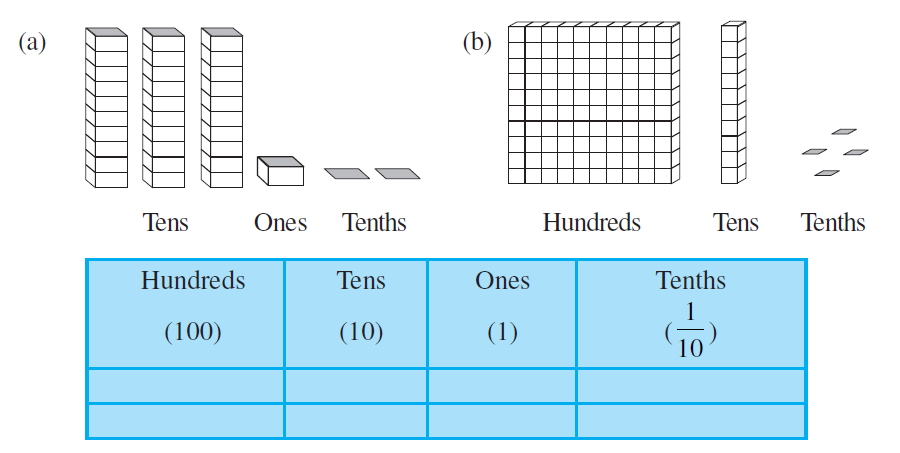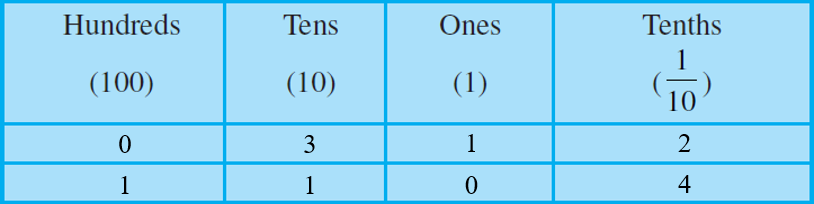Question 2: Write the following decimals in the place value table.
a) 19.4
b) 0.3
c) 10.6
d) 205.9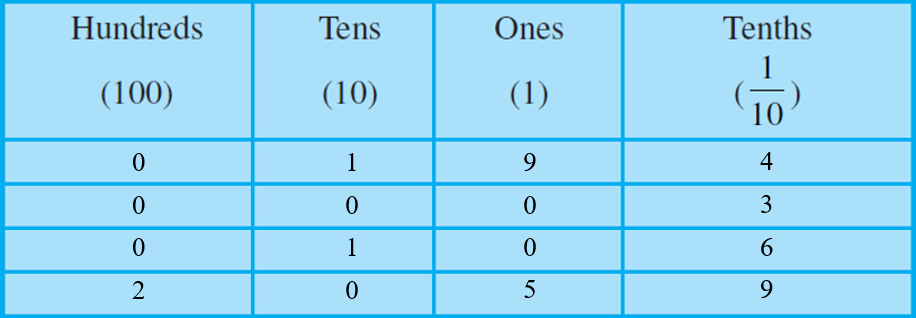Question 3: Write each of the following as decimals:
a) Seven-tenths
b) Two tens and nine-tenths
c) Fourteen point six
d) One hundred and two ones
e) Six hundred point eight

a) 7/10 = 0.7
b) 2 × 10 + 9/10 = 20.9
c) 14 + 0.6 = 14.6
d) 100 + 2 = 102.0
e) 600 + 0.8 = 600.8

Question 4: Write each of the following as decimals:
a) 5/10
b) 3 + 7/10
c) 200 + 60 + 5 + 1/10
d) 70 + 8/10
e) 88/10
f) 4 2/10
g) 3/2
h) 2/5
i) 12/5
j) 3 3/5
k) 4 1/2

a) 5/10 = 0.5

b) 3 + 7/10 = 3 + 0.7 = 3.7

c) 200 + 60 + 5 + 1/10 = 265 + 0.1 = 265.1

d) 70 + 8/10 = 70 + 0.8 = 70.8

e) 88/10 = 8.8

f) 4 2/10 = 4 × 10 + 2/10 = 42/10 = 4.2

g) 3/2 = 3/2 × 5/5 = 15/10 = 1.5

h) 2/5 = 2/5 × 2/2 = 4/10 = 0.4

i) 12/5 = 12/5 × 2/2 = 24/10 = 2.4

j) 3 3/5 = 3 × 5 + 3/5 = 18/5 = 18/5 × 2/2 = 36/10 = 3.6

k) 4 1/2 = 4 × 2 + 1/2 = 9/2 = 9/2 × 5/5 = 45/10 = 4.5

Question 5: Write the following decimals as fractions. Reduce the fractions to lowest form.
a) 0.6
b) 2.5
c) 1.0
d) 3.8
e) 13.7
f) 21.2
g) 6.4

a) 0.6 = 6/10 = 3/5

b) 2.5 = 25/10 = 5/2

c) 1.0 = 10/10 = 1

d) 3.8 = 38/10 = 19/5

e) 13.7 = 137/10

f) 21.2 = 212/10 = 106/5

g) 6.4 = 64/10 = 32/5

Question 6: Express the following as cm using decimals.
a) 2 mm
b) 30 mm
c) 116 mm
d) 4 cm 2 mm
e) 162 mm
f) 83 mm

a)
1 cm = 10 mm
2 mm = 2/10 cm
= 0.2 cm

b)
1 cm = 10 mm
30 mm = 30/10 cm
= 3.0 cm

c)
1 cm = 10 mm
116 mm = 116/10
= 11.6 cm

d)
1 cm = 2 mm
2 mm = 2/10 cm
= 0.2 cm
= 4 cm + 0.2 cm
= 4.2 cm

e)
1 cm = 10 mm
162 mm = 162/10
= 16.2 cm

f)
1 cm = 10 mm
83 mm = 83/10
= 8.3 cm

Question 7: Between which two whole numbers on the number line are the given numbers lie? Which of these whole numbers is nearer the number?
a) 0.8
b) 5.1
c) 2.6
d) 6.4
e) 9.1
f) 4.9

a) 0.8 lies between 0 and 1. 0.8 is more nearer to 1.

b) 5.1 lies between 5 and 6. 5.1 is more nearer to 5.

c) 2.6 lies between 2 and 3. 2.6 is more nearer to 3.

d) 6.4 lies between 6 and 7. 6.4 lies more nearer to 6.

e) 9.1 lies between 9 and 10. 9.1 lies more nearer to 9.

f) 4.9 lies between 4 and 5. 4.9 lies more nearer to 5.

Question 8: Show the following numbers on the number line.
a) 0.2
b) 1.9
c) 1.1
d) 2.5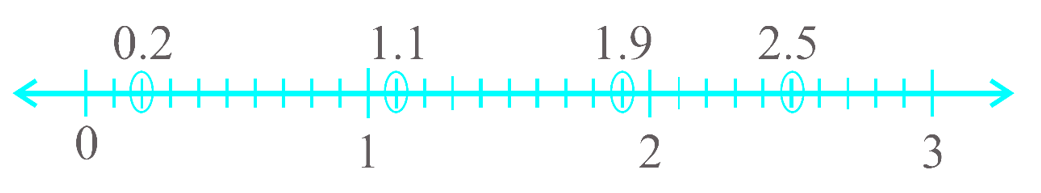Question 9: Write the decimal number represented by the points A, B, C, D on the given number line.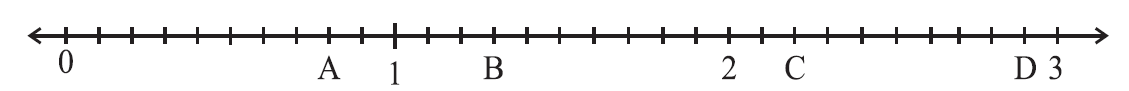A = 0.8
B = 1.3
C = 2.2
D = 2.9

Question 10:
a) The length of Ramesh’s notebook is 9 cm 5 mm. What will be its length in cm?
b) The length of a young gram plant is 65 mm. Express its length in cm.

a)
Length of Ramesh’s notebook = 9 cm 5 mm
Length of Ramesh’s notebook in cm = ?

1 cm = 10 mm
= 5 mm = 5/10 cm
= 0.5 cm
= 9 cm + 0.5 cm
= 9.5 cm

Therefore, the length of Ramesh’s notebook in cm is 9.5 cm.

b)
Length of young gram plant = 65 mm
Length of young gram plant in cm = ?

1 cm = 10 mm
= 65 mm = 65/10 cm
= 6.5 cm

Therefore, the length of young gram plant in cm is 6.5 cm.